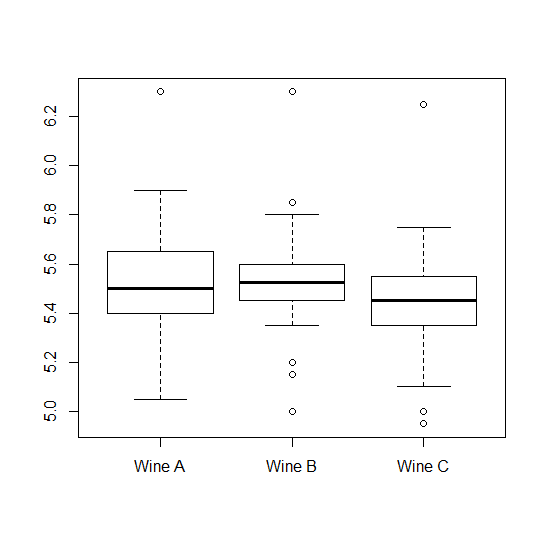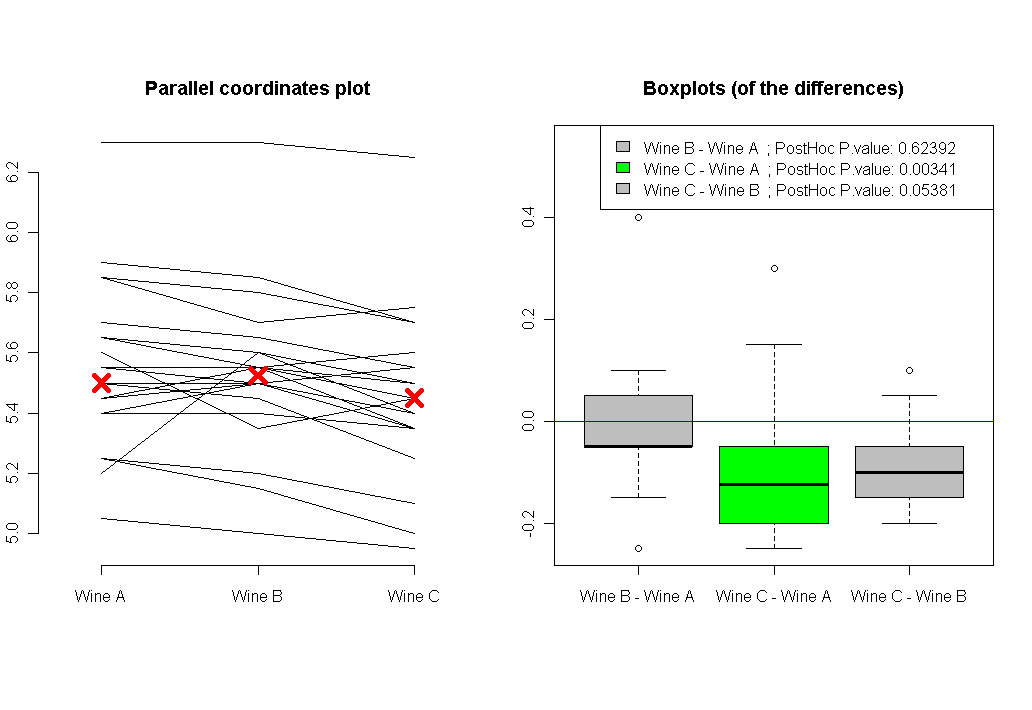## It’s not the p-values’ fault – reflections on the recent ASA statement (+relevant R resources)

Joint post by Yoav Benjamini and Tal Galili. The post highlights points raised by Yoav in his official response to the ASA statement (available as on page 4 in the ASA supplemental tab), as well as offers a list of relevant R resources.

### Summary

The ASA statement about the misuses of the p-value singles it out. It is just as well relevant to the use of most other statistical methods: context matters, no single statistical measure suffices, specific thresholds should be avoided and reporting should not be done selectively. The latter problem is discussed mainly in relation to omitted inferences. We argue that the selective reporting of inferences problem is serious enough a problem in our current industrialized science even when no omission takes place. Many R tools are available to address it, but they are mainly used in very large problems and are grossly underused in areas where lack of replicability hits hard.

## Post hoc analysis for Friedman's Test (R code)

My goal in this post is to give an overview of Friedman’s Test and then offer R code to perform post hoc analysis on Friedman’s Test results. (The R function can be downloaded from here)

### Preface: What is Friedman’s Test

Friedman test is a non-parametric randomized block analysis of variance. Which is to say it is a non-parametric version of a one way ANOVA with repeated measures. That means that while a simple ANOVA test requires the assumptions of a normal distribution and equal variances (of the residuals), the Friedman test is free from those restriction. The price of this parametric freedom is the loss of power (of Friedman’s test compared to the parametric ANOVa versions).

The hypotheses for the comparison across repeated measures are:

• H0: The distributions (whatever they are) are the same across repeated measures
• H1: The distributions across repeated measures are different

The test statistic for the Friedman’s test is a Chi-square with [(number of repeated measures)-1] degrees of freedom. A detailed explanation of the method for computing the Friedman test is available on Wikipedia.

Performing Friedman’s Test in R is very simple, and is by using the “friedman.test” command.

### Post hoc analysis for the Friedman’s Test

Assuming you performed Friedman’s Test and found a significant P value, that means that some of the groups in your data have different distribution from one another, but you don’t (yet) know which. Therefor, our next step will be to try and find out which pairs of our groups are significantly different then each other. But when we have N groups, checking all of their pairs will be to perform [n over 2] comparisons, thus the need to correct for multiple comparisons arise.
Our first task will be to perform a post hoc analysis of our results (using R) – in the hope of finding out which of our groups are responsible that we found that the null hypothesis was rejected. While in the simple case of ANOVA, an R command is readily available (“TukeyHSD”), in the case of friedman’s test (until now) the code to perform the post hoc test was not as easily accessible.
Our second task will be to visualize our results. While in the case of simple ANOVA, a boxplot of each group is sufficient, in the case of a repeated measures – a boxplot approach will be misleading to the viewer. Instead, we will offer two plots: one of parallel coordinates, and the other will be boxplots of the differences between all pairs of groups (in this respect, the post hoc analysis can be thought of as performing paired wilcox.test with correction for multiplicity).

### R code for Post hoc analysis for the Friedman’s Test

The analysis will be performed using the function (I wrote) called “friedman.test.with.post.hoc”, based on the packages “coin” and “multcomp”. Just a few words about it’s arguments:

• formu – is a formula object of the shape: Y ~ X | block (where Y is the ordered (numeric) responce, X is a group indicator (factor), and block is the block (or subject) indicator (factor)
• data – is a data frame with columns of Y, X and block (the names could be different, of course, as long as the formula given in “formu” represent that)
• All the other parameters are to allow or suppress plotting of the results.
```friedman.test.with.post.hoc <- function(formu, data, to.print.friedman = T, to.post.hoc.if.signif = T,  to.plot.parallel = T, to.plot.boxplot = T, signif.P = .05, color.blocks.in.cor.plot = T, jitter.Y.in.cor.plot =F)
{
# formu is a formula of the shape: 	Y ~ X | block
# data is a long data.frame with three columns:    [[ Y (numeric), X (factor), block (factor) ]]

# Note: This function doesn't handle NA's! In case of NA in Y in one of the blocks, then that entire block should be removed.

if(!require(coin))
{
print("You are missing the package 'coin', we will now try to install it...")
install.packages("coin")
library(coin)
}

if(!require(multcomp))
{
print("You are missing the package 'multcomp', we will now try to install it...")
install.packages("multcomp")
library(multcomp)
}

if(!require(colorspace))
{
print("You are missing the package 'colorspace', we will now try to install it...")
install.packages("colorspace")
library(colorspace)
}

# get the names out of the formula
formu.names <- all.vars(formu)
Y.name <- formu.names
X.name <- formu.names
block.name <- formu.names

if(dim(data) >3) data <- data[,c(Y.name,X.name,block.name)]	# In case we have a "data" data frame with more then the three columns we need. This code will clean it from them...

# Note: the function doesn't handle NA's. In case of NA in one of the block T outcomes, that entire block should be removed.

# stopping in case there is NA in the Y vector
if(sum(is.na(data[,Y.name])) > 0) stop("Function stopped: This function doesn't handle NA's. In case of NA in Y in one of the blocks, then that entire block should be removed.")

# make sure that the number of factors goes with the actual values present in the data:
data[,X.name ] <- factor(data[,X.name ])
data[,block.name ] <- factor(data[,block.name ])
number.of.X.levels <- length(levels(data[,X.name ]))
if(number.of.X.levels == 2) { warning(paste("'",X.name,"'", "has only two levels. Consider using paired wilcox.test instead of friedman test"))}

# making the object that will hold the friedman test and the other.
the.sym.test <- symmetry_test(formu, data = data,	### all pairwise comparisons
teststat = "max",
xtrafo = function(Y.data) { trafo( Y.data, factor_trafo = function(x) { model.matrix(~ x - 1) %*% t(contrMat(table(x), "Tukey")) } ) },
ytrafo = function(Y.data){ trafo(Y.data, numeric_trafo = rank, block = data[,block.name] ) }
)
# if(to.print.friedman) { print(the.sym.test) }

if(to.post.hoc.if.signif)
{
if(pvalue(the.sym.test) < signif.P)
{
# the post hoc test
The.post.hoc.P.values <- pvalue(the.sym.test, method = "single-step")	# this is the post hoc of the friedman test

# plotting
if(to.plot.parallel & to.plot.boxplot)	par(mfrow = c(1,2)) # if we are plotting two plots, let's make sure we'll be able to see both

if(to.plot.parallel)
{
X.names <- levels(data[, X.name])
X.for.plot <- seq_along(X.names)
plot.xlim <- c(.7 , length(X.for.plot)+.3)	# adding some spacing from both sides of the plot

if(color.blocks.in.cor.plot)
{
blocks.col <- rainbow_hcl(length(levels(data[,block.name])))
} else {
blocks.col <- 1 # black
}

data2 <- data
if(jitter.Y.in.cor.plot) {
data2[,Y.name] <- jitter(data2[,Y.name])
par.cor.plot.text <- "Parallel coordinates plot (with Jitter)"
} else {
par.cor.plot.text <- "Parallel coordinates plot"
}

# adding a Parallel coordinates plot
matplot(as.matrix(reshape(data2,  idvar=X.name, timevar=block.name,
direction="wide")[,-1])  ,
type = "l",  lty = 1, axes = FALSE, ylab = Y.name,
xlim = plot.xlim,
col = blocks.col,
main = par.cor.plot.text)
axis(1, at = X.for.plot , labels = X.names) # plot X axis
axis(2) # plot Y axis
points(tapply(data[,Y.name], data[,X.name], median) ~ X.for.plot, col = "red",pch = 4, cex = 2, lwd = 5)
}

if(to.plot.boxplot)
{
# first we create a function to create a new Y, by substracting different combinations of X levels from each other.
subtract.a.from.b <- function(a.b , the.data)
{
the.data[,a.b] - the.data[,a.b]
}

temp.wide <- reshape(data,  idvar=X.name, timevar=block.name,
direction="wide") 	#[,-1]
wide.data <- as.matrix(t(temp.wide[,-1]))
colnames(wide.data) <- temp.wide[,1]

Y.b.minus.a.combos <- apply(with(data,combn(levels(data[,X.name]), 2)), 2, subtract.a.from.b, the.data =wide.data)
names.b.minus.a.combos <- apply(with(data,combn(levels(data[,X.name]), 2)), 2, function(a.b) {paste(a.b,a.b,sep=" - ")})

the.ylim <- range(Y.b.minus.a.combos)
the.ylim <- the.ylim + max(sd(Y.b.minus.a.combos))	# adding some space for the labels
is.signif.color <- ifelse(The.post.hoc.P.values < .05 , "green", "grey")

boxplot(Y.b.minus.a.combos,
names = names.b.minus.a.combos ,
col = is.signif.color,
main = "Boxplots (of the differences)",
ylim = the.ylim
)
legend("topright", legend = paste(names.b.minus.a.combos, rep(" ; PostHoc P.value:", number.of.X.levels),round(The.post.hoc.P.values,5)) , fill =  is.signif.color )
abline(h = 0, col = "blue")

}

list.to.return <- list(Friedman.Test = the.sym.test, PostHoc.Test = The.post.hoc.P.values)
if(to.print.friedman) {print(list.to.return)}
return(list.to.return)

}	else {
print("The results where not significant, There is no need for a post hoc test")
return(the.sym.test)
}
}

# Original credit (for linking online, to the package that performs the post hoc test) goes to "David Winsemius", see:
# http://tolstoy.newcastle.edu.au/R/e8/help/09/10/1416.html
}

```

### Example

(The code for the example is given at the end of the post)

Let's make up a little story: let's say we have three types of wine (A, B and C), and we would like to know which one is the best one (in a scale of 1 to 7). We asked 22 friends to taste each of the three wines (in a blind fold fashion), and then to give a grade of 1 till 7 (for example sake, let's say we asked them to rate the wines 5 times each, and then averaged their results to give a number for a persons preference for each wine. This number which is now an average of several numbers, will not necessarily be an integer).

After getting the results, we started by performing a simple boxplot of the ratings each wine got. Here it is:The plot shows us two things: 1) that the assumption of equal variances here might not hold. 2) That if we are to ignore the "within subjects" data that we have, we have no chance of finding any difference between the wines.

So we move to using the function "friedman.test.with.post.hoc" on our data, and we get the following output:

\$Friedman.Test
Asymptotic General Independence Test
data:  Taste by
Wine (Wine A, Wine B, Wine C)
stratified by Taster
maxT = 3.2404, p-value = 0.003421
\$PostHoc.Test
Wine B - Wine A 0.623935139
Wine C - Wine A 0.003325929
Wine C - Wine B 0.053772757

The conclusion is that once we take into account the within subject variable, we discover that there is a significant difference between our three wines (significant P value of about  0.0034). And the posthoc analysis shows us that the difference is due to the difference in tastes between Wine C and Wine A (P value 0.003). and maybe also with the difference between Wine C and Wine B (the P value is 0.053, which is just borderline significant).

Plotting our analysis will also show us the direction of the results, and the connected answers of each of our friends answers:Here is the code for the example:

```source("https://www.r-statistics.com/wp-content/uploads/2010/02/Friedman-Test-with-Post-Hoc.r.txt")  # loading the friedman.test.with.post.hoc function from the internet

### Comparison of three Wine ("Wine A", "Wine B", and
###  "Wine C") for rounding first base.
WineTasting <- data.frame(
Taste = c(5.40, 5.50, 5.55,
5.85, 5.70, 5.75,
5.20, 5.60, 5.50,
5.55, 5.50, 5.40,
5.90, 5.85, 5.70,
5.45, 5.55, 5.60,
5.40, 5.40, 5.35,
5.45, 5.50, 5.35,
5.25, 5.15, 5.00,
5.85, 5.80, 5.70,
5.25, 5.20, 5.10,
5.65, 5.55, 5.45,
5.60, 5.35, 5.45,
5.05, 5.00, 4.95,
5.50, 5.50, 5.40,
5.45, 5.55, 5.50,
5.55, 5.55, 5.35,
5.45, 5.50, 5.55,
5.50, 5.45, 5.25,
5.65, 5.60, 5.40,
5.70, 5.65, 5.55,
6.30, 6.30, 6.25),
Wine = factor(rep(c("Wine A", "Wine B", "Wine C"), 22)),
Taster = factor(rep(1:22, rep(3, 22))))

with(WineTasting , boxplot( Taste  ~ Wine )) # boxploting
friedman.test.with.post.hoc(Taste ~ Wine | Taster ,WineTasting)	# the same with our function. With post hoc, and cool plots
```

If you find this code useful, please let me know (in the comments) so I will know there is a point in publishing more such code snippets...